Question

A conducting sphere of radius R1 carries a charge Q. Another conducting sphere has a radius R2 = 3 4 R1, but carries the same charge. The spheres are far apart. What is the ratio E2 E1 of the electric field near the surface of the sphere with radius R2 to the field near the surface of the sphere with radius R1?

1.RuslanHeatt

The ratio of electric field is 16:9.

Explanation:

Given that,

Radius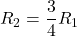Charge = Q

We know that,

The electric field is directly proportional to the charge and inversely proportional to the square of the distance.

In mathematically term,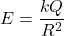Here,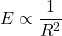We need to calculate the ratio of electric field

Using formula of electric field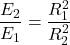Put the value into the formula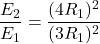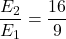Hence, The ratio of electric field is 16:9.